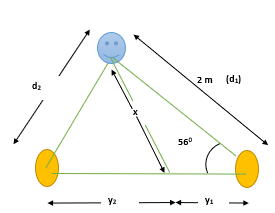A loudspeaker A and point C, where a listener is positioned. |AC| = 2.00 m and the angle theta =...

Question:

A loudspeaker A and point C, where a listener is positioned. |AC| = 2.00 m and the angle {eq}\theta {/eq} = 56{eq}^{\circ} {/eq}. A second loudspeaker B is located somewhere to the left of A. The speakers vibrate out of phase and are playing a 60.0 Hz tone. The speed of sound is 343 m/s.

What is the second closest distance to speaker A that speaker B can be located, so that the listener hears no sound?

Sound Interference:

The sound waves travel in the form of waves so the cancellation of the waves (interference) will occur when the path difference occurs in the multiples of half odd integral.

The visualization of the problem can be clearly understood by the following diagram,Condition for destructive interference is represented as,

{eq}d_2 - d_1 = \dfrac{n \lambda}{2}\;\;\; n= 1,3,5...\\ \textrm{where,}\\ \lambda = \dfrac{343}{60} = 5.71\; \textrm{m}\\ \textrm{Therefore, the path difference becomes,}\\ d_2 = \dfrac{(1)(5.71)}{2}+2\; (\because d_1 = 2\; \textrm{m})\\ d_2 = 4.855\; \textrm{m} {/eq}

Using the trigonometric identities we compute,

{eq}y_1 = d_1 \cos 56° = (2)(0.56) = 1.12\; \textrm{m}\\ x = d_1 \sin56° = (2)(0.83) = 1.66\; \textrm{m}\\ \textrm{Therefore,}\; y_2\; \textrm{becomes,}\\ y_2 =\sqrt{d^2_2 - x^2}\\ y_2 = \sqrt{23.57 - 2.75} = 4.56\; \textrm{m}\\ {/eq}

Therefore, summing the computed values of the {eq}y_1 \; \textrm{&}\; y_2 {/eq} we get,

{eq}\Rightarrow y_1+y_2 = 4.56 + 1.12 = 5.68\; \textrm{m} {/eq}

Thus, 5.68 m is the required distance of speaker B from speaker A such that the observer at point C will hear no sound.